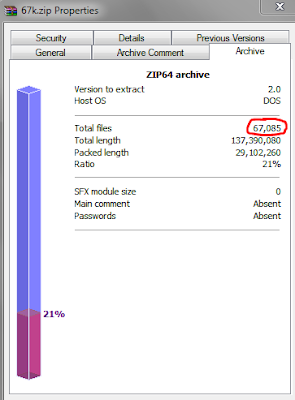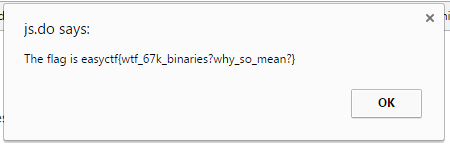## Sunday, 26 March 2017

### 67,000 cuts with python-pefile

EasyCTF featured an interesting reversing engineering challenge. The problem statement is shown in Figure 1.Figure 1: Problem statement
A file 67k.zip was provided containing 67,085 PE files numbered from 00000.exe to 1060c.exe as shown in Figure 2.Figure 2: 67k files to reverse!

The task was to reverse engineer each of them and combine their solutions to get the flag. All of the files were exactly 2048 bytes in size as shown in Figure 3.Figure 3: 2048 all the way
Let's analyze one of the files, say the first one 00000.exe in IDA. The graph view is simple as in Figure 4.Figure 4: Graph view of 00000.exe
The program accepts one integer input through scanf. This is compared with another number generated by a simple operation like sub on two hard-coded integers stored in register eax and ecx. If they match, we go to the green basic block on the left. It does another calculation (sar - Shift Arithmetic Right at 402042) and finally prints this calculated value along with the success message at 40204F. This general pattern is followed by all of the 67,085 files with minor changes as enumerated below:

• The imagebase and the entrypoint of the PE vary with each file.
• The operation on the two hardcoded integers can be any of addition, subtraction or xor.
• The address of the function (op_sub in the example) performing the operation varies.
• The address of the hard coded integer (dword_403000 in the example) varies.
• The amount of shift stored in byte_403007 also varies.

Obviously, reversing 67k files by hand is not possible and requires automation. For this task, I choose the pefile module by Ero Carrera. First, we need to get the offsets of the individual instructions from the Entry point. We can do this from OllyDbg as in the following listing. The offsets are in the left most column.

``````<Modul>/\$  68 5E304000       push 0040305E                            ; /s = "Launch codes?"
\$+5   >|.  FF15 44104000     call dword ptr [<&msvcrt.puts>]          ; \puts
\$+B   >|.  58                pop eax
\$+C   >|.  68 6C304000       push 0040306C
\$+11  >|.  68 04304000       push 00403004                            ; /format = "%d"
\$+16  >|.  FF15 48104000     call dword ptr [<&msvcrt.scanf>]         ; \scanf
\$+1C  >|.  83C4 08           add esp,8
\$+1F  >|.  A1 00304000       mov eax,dword ptr 
\$+24  >|.  B9 EDA7A8A1       mov ecx,A1A8A7ED
\$+29  >|.  E8 CFFFFFFF       call <op_sub>
\$+2E  >|.  3B05 6C304000     cmp eax,dword ptr [40306C]
\$+34  >|.  75 1E             jnz short 0040205A
\$+36  >|.  8A0D 07304000     mov cl,byte ptr 
\$+3C  >|.  D3F8              sar eax,cl
\$+3E  >|.  25 FF000000       and eax,0FF
\$+43  >|.  50                push eax                                 ; /<%c>
\$+44  >|.  68 34304000       push 00403034                            ; |format = "Wow you got it. Here is the result: (%c)"
\$+49  >|.  FF15 4C104000     call dword ptr [<&msvcrt.printf>]        ; \printf
\$+4F  >|.  83C4 08           add esp,8
\$+52  >|.  EB 0C             jmp short 00402066
\$+54  >|>  68 08304000       push 00403008                            ; /s = "I think my dog figured this out before you."
\$+59  >|.  FF15 44104000     call dword ptr [<&msvcrt.puts>]          ; \puts
\$+5F  >|.  58                pop eax
\$+60  >\>  C3                ret
``````

The complete script is provided below.
``````import zipfile
import struct
import pefile
import cStringIO

def rshift(val, n):
"""
Implements arithmetic right shift on 32 bits
"""
return (val % 0x100000000) >> n

def process(buf):
# Load the Pe file
pe = pefile.PE(data=buf, fast_load=True)

# RVA of Entry Point

# \$+1F  >|.  A1 00304000       mov eax,dword ptr 
# \$+24  >|.  B9 EDA7A8A1       mov ecx,A1A8A7ED
eax = pe.get_dword_at_rva(pe.get_dword_at_rva(ep + 0x1f + 1) - imagebase)
ecx = pe.get_dword_at_rva(ep + 0x24 + 1)

# \$+29  >|.  E8 CFFFFFFF       call <op_sub>
fn_offs = struct.unpack('<i', pe.get_data(ep + 0x29 + 1, length = 4))

# function rva = instruction address + length + func offset from imagebase
fn_rva = 0x29 + 5 + fn_offs

# Get the first byte of the function (op_sub)
func_byte = ord(pe.get_data(rva = ep+fn_rva, length=1))

# Perform the operation based on the function byte

# op_xor
# 31C8            xor eax,ecx
# C3              ret
if func_byte == 0x31:
eax ^= ecx

# 01C8            add eax,ecx
# C3              ret
elif func_byte == 0x1:
eax += ecx

# op_sub
# 29C8            sub eax,ecx
# C3              ret
elif func_byte == 0x29:
eax -= ecx

else:
raise 'Error'

# \$+36  >|.  8A0D 07304000     mov cl,byte ptr 
# \$+3C  >|.  D3F8              sar eax,cl
# \$+3E  >|.  25 FF000000       and eax,0FF
cl = ord(pe.get_data(pe.get_dword_at_rva(ep+0x36+2)-imagebase, 1))

return chr(rshift(eax, cl) & 0xFF)

if __name__ == '__main__':
output = cStringIO.StringIO()
with zipfile.ZipFile('67k.zip') as f:
for idx in xrange(67085):
fname = format(idx, 'x').zfill(5) + '.exe'
output.write(process(buf))
# Fast divisiblity check by 1024, 2^10 (last 10 bits must be zero)
if idx & 0x3FF == 0:
print 'Completed', idx

open('output.txt', 'w').write(output.getvalue())
print 'Done!!'
``````
Instead of unpacking 67,085 files to the hard drive and fragmenting it in the process, I have used the zipfile module to access the files within the archive. However, zipfile throws an error on opening the archive and must be modified slightly as described in this Stack Overflow answer.

We access the instructions by using the offsets from the entry point. The address of the operation function and the values of the hard-coded integers, shift amount are also obtained similarly. We discern the type of operation performed by examining its first byte. With this information, we can find the correct output.

Running the script on stock Python 2.7 takes close to 15 minutes. With PyPy, this is reduced to 2 minutes. We get a 66 kb output consisting of obfuscated javascript as shown in Figure 5.Figure 5: Obfuscated javascript output
Running the obfuscated javascript on jsfiddle gives us the flag easyctf{wtf_67k_binaries?why_so_mean?} as also shown in Figure 6:Figure 6: Finally we get the flag